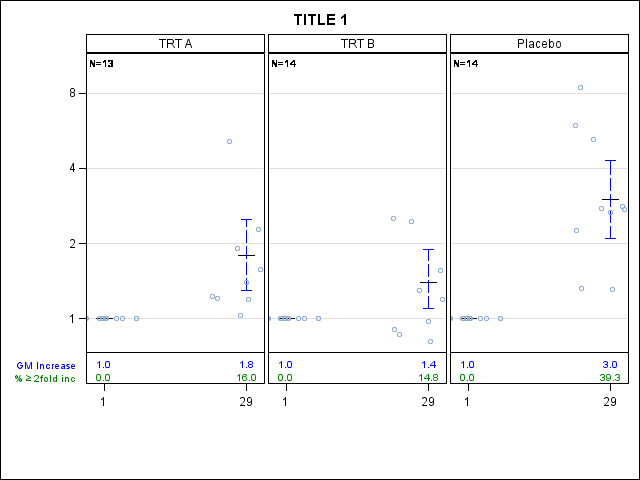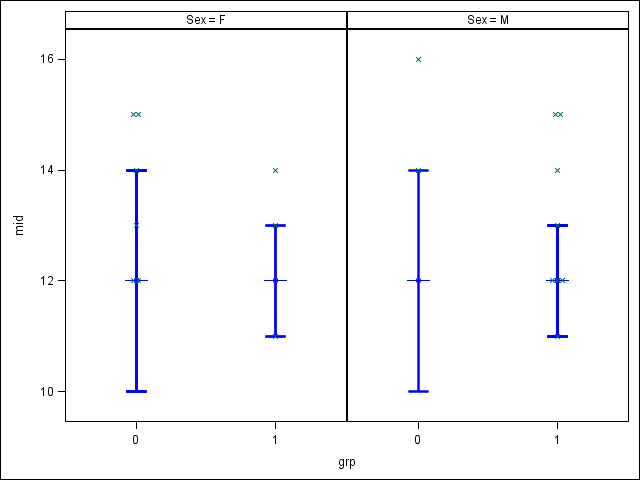## Jitter within Sgpanel

Dear All,

I'm using sgpanel procedure of SAS 9.4 to get a graph.

I have 2 days (1 and 29) and 3 trt (TRT A, TRT B and Placebo).

my sas code used :

``````PROC FORMAT ;
VALUE dy  1    = '1 '
29='29'
OTHER =' ';
value trtn 1="TRT A"
2="TRT B"
3='Placebo';
RUN;

PROC SGPANEL DATA=try noautolegend pad=( bottom=10%);
title "TITLE 1";

FORMAT trtgpn trtn.;
PANELBY TRTGPN   / columns=3 LAYOUT=COLUMNLATTICE  sparse SPACING=3

HIGHLOW y=emn low=lo high=hi/lineattrs=(thickness=1.7 color=black) type=line ;
HIGHLOW x=vis low=lclm high=uclm/lineattrs=(thickness=1.7 color=blue) type=line LOWCAP=SERIF HIGHCAP=SERIF;
SCATTER X=xc Y=yc/ markerchar=nbc MARKERCHARATTRS=(size=7 color=black )  ;
SCATTER X=vis Y=ratio/jitter jitterwidth=0.50 markerattrs=(size=8 color=VLIGB symbol=circle ) ;

COLAXIS label=' ' TICKVALUEFORMAT=dy.  values=( 1 29 ) INTEGER ;
ROWAXIS type=log logbase=2 grid display=(nolabel) ;

COLAXISTABLE emnx / x=vis valueattrs=(color=blue)labelattrs=(color=blue size=7) label="GM Increase" SEPARATOR;
COLAXISTABLE perc / x=vis valueattrs=(color=green) labelattrs=(color=green size=7)
label= "% (*ESC*){unicode '2265'x} 2fold inc";
RUN;``````

my probelem is with option Jitter that not work for day 29  (on day 29 i don't have any jittering point) but in my graph i have results of day 29 scatter around 29!!! see results bellow :other problem : the line presenting high low points (in blue)  is cutted in some point !! and the line (in black) representing mean of day 1 are behind scatter points!!

1 ACCEPTED SOLUTION

Accepted SolutionsPGStats
Opal | Level 21

## Re: Jitter within Sgpanel

Making colaxis a type=discrete axis would probably help

``````data g;
set sashelp.class;
grp = rand("uniform") < 0.5;
if grp then do;
mid=12; low=11; high=13; end;
else do;
mid=12; low=10; high=14; end;
run;

proc sgpanel data=g noautolegend;
panelby sex / rows=1;
scatter x=grp y=mid /
yerrorlower=low yerrorupper=high errorbarattrs=(thickness=2 color=blue);
scatter x=grp y=mid / markerattrs=(symbol=Plus size=30 color=blue);
scatter x=grp y=age / jitter;
colaxis type=discrete;
run;``````PG
2 REPLIES 2PGStats
Opal | Level 21

## Re: Jitter within Sgpanel

Making colaxis a type=discrete axis would probably help

``````data g;
set sashelp.class;
grp = rand("uniform") < 0.5;
if grp then do;
mid=12; low=11; high=13; end;
else do;
mid=12; low=10; high=14; end;
run;

proc sgpanel data=g noautolegend;
panelby sex / rows=1;
scatter x=grp y=mid /
yerrorlower=low yerrorupper=high errorbarattrs=(thickness=2 color=blue);
scatter x=grp y=mid / markerattrs=(symbol=Plus size=30 color=blue);
scatter x=grp y=age / jitter;
colaxis type=discrete;
run;``````PG

## Re: Jitter within Sgpanel

Thanks PGstats..it works.

Discussion stats
• 2 replies
• 1752 views
• 0 likes
• 2 in conversation